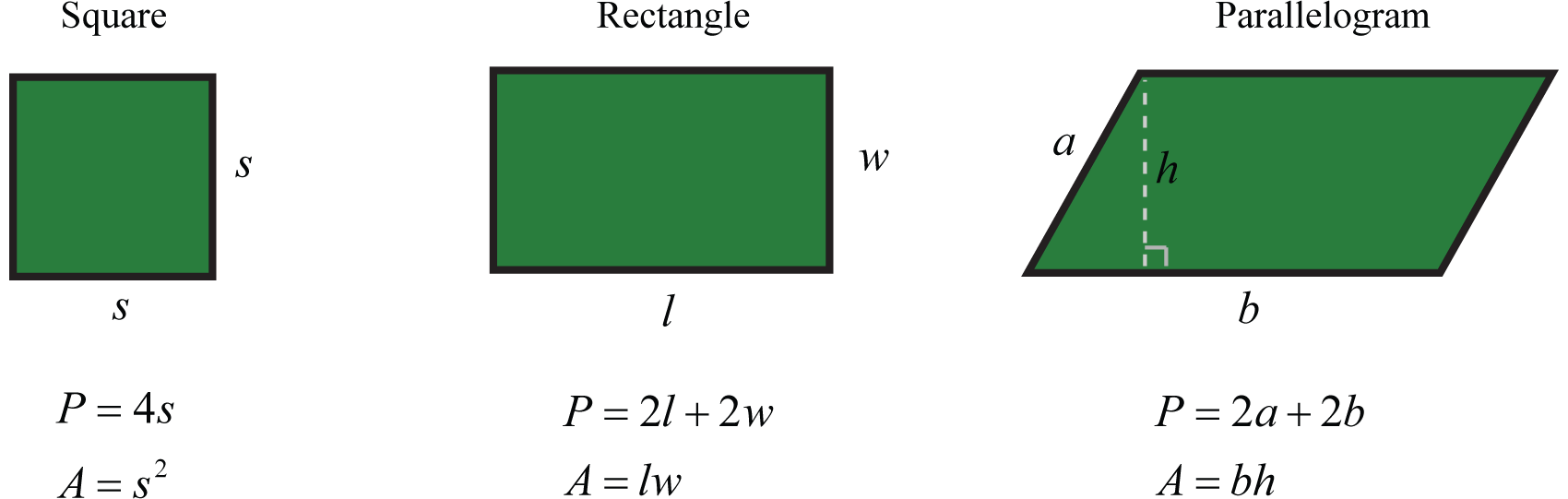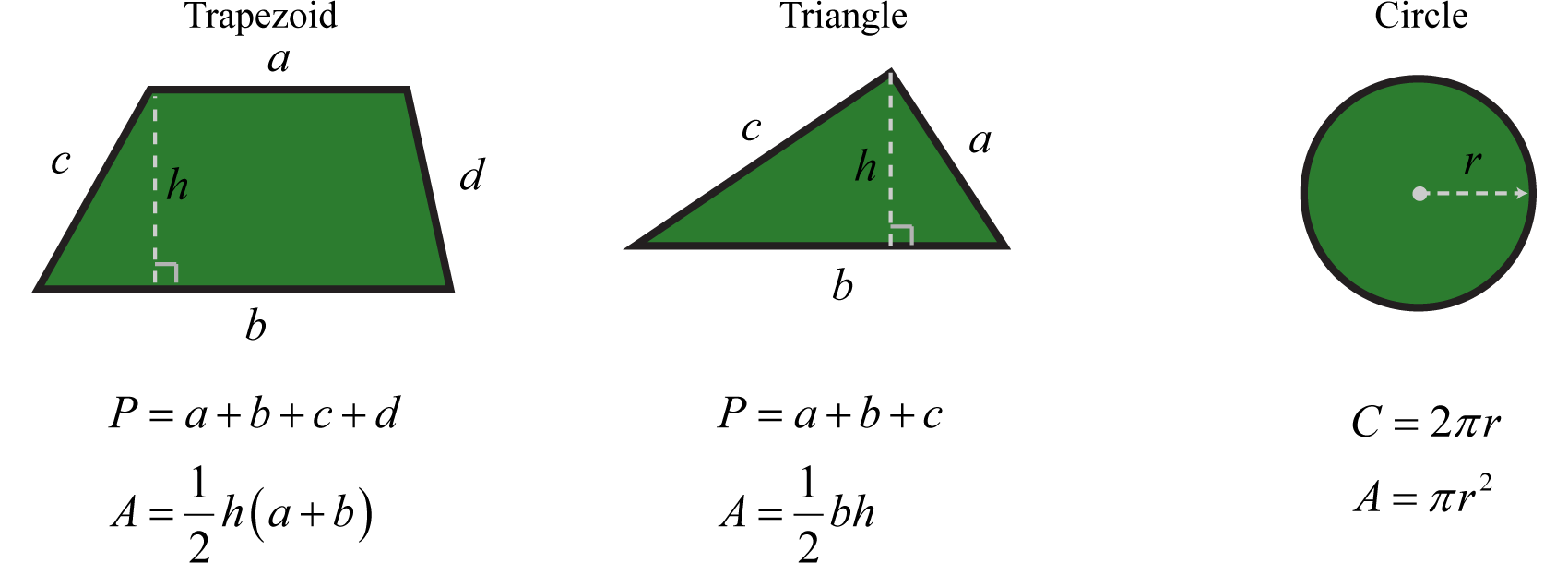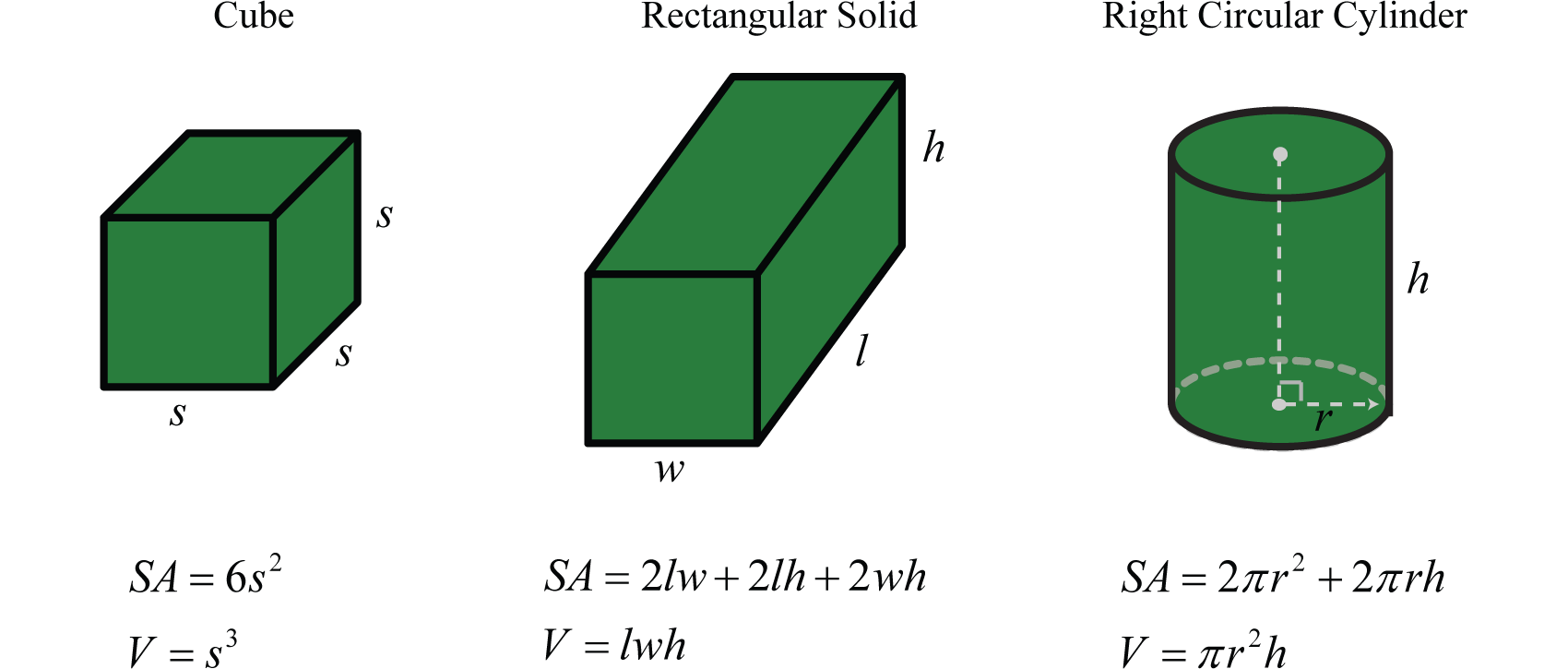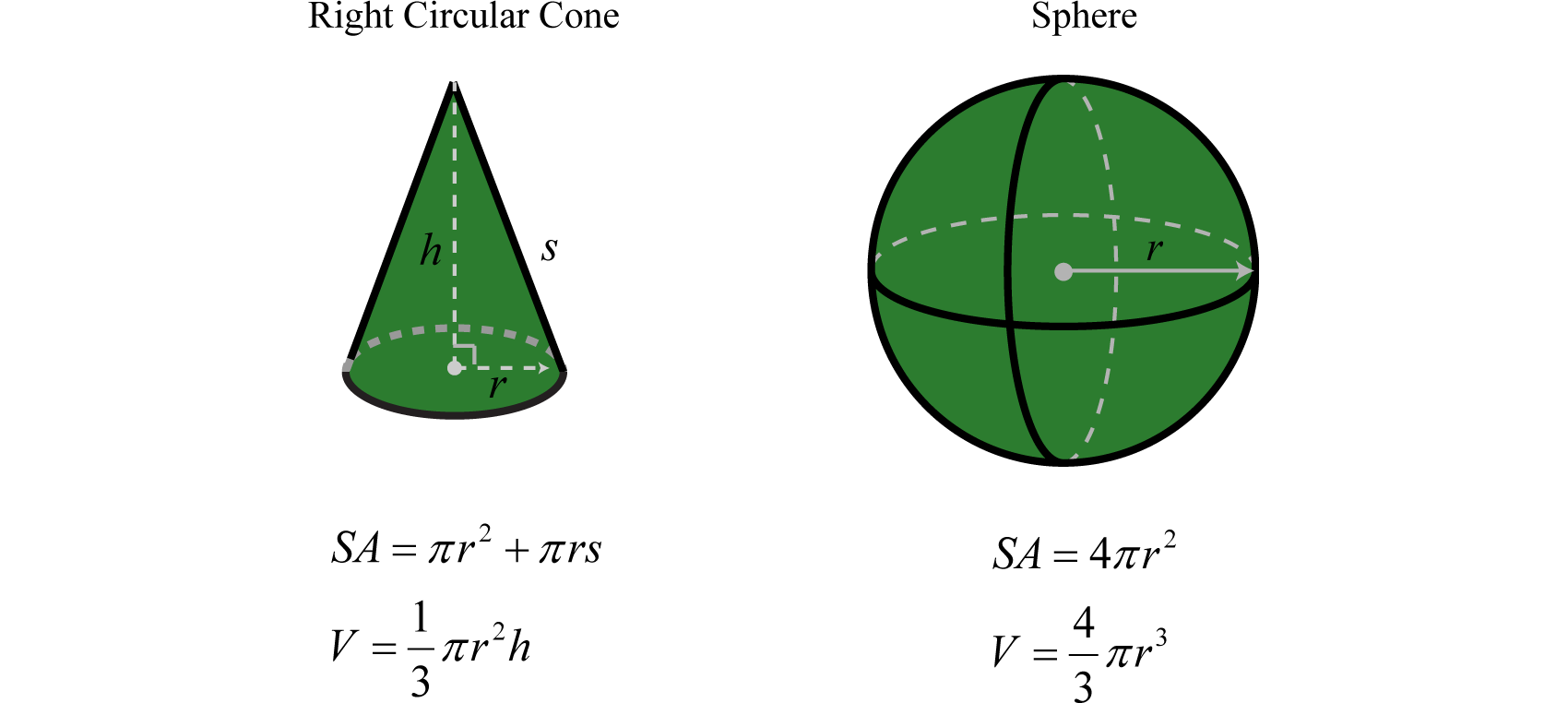This is “Algebraic Expressions and Formulas”, section 1.4 from the book Advanced Algebra (v. 1.0). For details on it (including licensing), click here.

Has this book helped you? Consider passing it on:
Creative Commons supports free culture from music to education. Their licenses helped make this book available to you.
DonorsChoose.org helps people like you help teachers fund their classroom projects, from art supplies to books to calculators.

## 1.4 Algebraic Expressions and Formulas

### Learning Objectives

1. Identify the parts of an algebraic expression.
2. Apply the distributive property.
3. Evaluate algebraic expressions.
4. Use formulas that model common applications.

## Algebraic Expressions and the Distributive Property

In algebra, letters called variables are used to represent numbers. Combinations of variables and numbers along with mathematical operations form algebraic expressionsCombinations of variables and numbers along with mathematical operations used to generalize specific arithmetic operations., or just expressions. The following are some examples of expressions with one variable, $x$:

 $2x+3$ $x2−9$ $1x+xx+2$ $3x+x$

TermsComponents of an algebraic expression separated by addition operators. in an algebraic expression are separated by addition operators and factorsComponents of a term separated by multiplication operators. are separated by multiplication operators. The numerical factor of a term is called the coefficientThe numerical factor of a term.. For example, the algebraic expression $x2y2+6xy−3$ can be thought of as $x2y2+6xy+(−3)$ and has three terms. The first term, $x2y2$, represents the quantity $1x2y2=1⋅x⋅x⋅y⋅y$ where 1 is the coefficient and x and y are the variables. All of the variable factors with their exponents form the variable part of a termAll the variable factors with their exponents.. If a term is written without a variable factor, then it is called a constant termA term written without a variable factor.. Consider the components of $x2y2+6xy−3$,

Terms

Coefficient

Variable Part

$x2y2$

1

$x2y2$

$6xy$

6

$xy$

−3

−3

The third term in this expression, −3, is called a constant term because it is written without a variable factor. While a variable represents an unknown quantity and may change, the constant term does not change.

### Example 1

List all coefficients and variable parts of each term: $10a2−5ab−b2.$

Solution:

We want to think of the third term in this example $−b2$ as $−1b2.$

Terms

Coefficient

Variable Part

$10a2$

10

$a2$

$−5ab$

−5

$ab$

$−b2$

−1

$b2$

Answer: Coefficients: ${−5, −1, 10}$; Variable parts: ${a2, ab, b2}$

In our study of algebra, we will encounter a wide variety of algebraic expressions. Typically, expressions use the two most common variables, x and y. However, expressions may use any letter (or symbol) for a variable, even Greek letters, such as alpha ($α$) and beta ($β$). Some letters and symbols are reserved for constants, such as $π≈3.14159$ and $e≈2.71828.$ Since there is only a limited number of letters, you will also use subscripts,$x1, x2, x3, x4,…$, to indicate different variables.

The properties of real numbers are important in our study of algebra because a variable is simply a letter that represents a real number. In particular, the distributive propertyGiven any real numbers a, b, and c, $a(b+c)=ab+ac$ or $(b+c)a=ba+ca.$ states that if given any real numbers a, b and c, then,

$a(b+c)=ab+ac$

This property is one that we apply often when simplifying algebraic expressions. To demonstrate how it will be used, we simplify $2(5−3)$ in two ways, and observe the same correct result.

Working parenthesis first.

Using the distributive property.

$2(5−3)=2(2)=4$

$2(5−3)=2⋅5−2⋅3=10−6=4$

Certainly, if the contents of the parentheses can be simplified we should do that first. On the other hand, when the contents of parentheses cannot be simplified any further, we multiply every term within it by the factor outside of it using the distributive property. Applying the distributive property allows us to multiply and remove the parentheses.

### Example 2

Simplify: $5(−2a+5b)−2c.$

Solution:

Multiply only the terms grouped within the parentheses for which we are applying the distributive property.$=5⋅(−2a)+5⋅5b−2c=−10a+25b−2c$

Answer: $−10a+25b−2c$

Recall that multiplication is commutative and therefore we can write the distributive property in the following manner, $(b+c)a=ba+ca.$

### Example 3

Simplify: $(3x−4y+1)⋅3.$

Solution:

Multiply all terms within the parenthesis by 3.

$(3x−4y+1)⋅3=3x⋅3−4y⋅3+1⋅3=9x−12y+3$

Answer:$9x−12y+3$

Terms whose variable parts have the same variables with the same exponents are called like termsConstant terms or terms whose variable parts have the same variables with the same exponents., or similar termsUsed when referring to like terms.. Furthermore, constant terms are considered to be like terms. If an algebraic expression contains like terms, apply the distributive property as follows:

$5x+7x=(5+7)x=12x4x2+5x2−7x2=(4+5−7)x2=2x2$

In other words, if the variable parts of terms are exactly the same, then we can add or subtract the coefficients to obtain the coefficient of a single term with the same variable part. This process is called combining like termsAdding or subtracting like terms within an algebraic expression to obtain a single term with the same variable part.. For example,

$12x2y3+3x2y3=15x2y3$

Notice that the variable factors and their exponents do not change. Combining like terms in this manner, so that the expression contains no other similar terms, is called simplifying the expressionThe process of combining like terms until the expression contains no more similar terms.. Use this idea to simplify algebraic expressions with multiple like terms.

### Example 4

Simplify: $x2−10x+8+5x2−6x−1.$

Solution:

Identify the like terms and add the corresponding coefficients.

$1x2–− 10x ––+ 8 –––+ 5x2–− 6x ––− 1 ––– Combine like terms.=6x2−16x+7$

Answer: $6x2−16x+7$

### Example 5

Simplify: $a2b2−ab−2(2a2b2−5ab+1).$

Solution:

Distribute −2 and then combine like terms.

$a2b2−ab−2(2a2b2−5ab+1)=a2b2−ab−4a2b2+10ab−2=−3a2b2+9ab−2$

Answer: $−3a2b2+9ab−2$

## Evaluating Algebraic Expressions

An algebraic expression can be thought of as a generalization of particular arithmetic operations. Performing these operations after substituting given values for variables is called evaluatingThe process of performing the operations of an algebraic expression for given values of the variables.. In algebra, a variable represents an unknown value. However, if the problem specifically assigns a value to a variable, then you can replace that letter with the given number and evaluate using the order of operations.

### Example 6

Evaluate:

1. $5x−2$ where $x=23$
2. $y2−y−6$ where $y=−4$

Solution:

To avoid common errors, it is a best practice to first replace all variables with parentheses, and then replace, or substituteThe act of replacing a variable with an equivalent quantity., the appropriate given value.

a.

$5x−2=5( )−2=5(23)−2=103−21⋅33=10−63=43$

b.

$y2−y−6=( )2−( )−6=(−4)2−(−4)−6=16+4−6=14$

1. $43$
2. 14

Often algebraic expressions will involve more than one variable.

### Example 7

Evaluate $a3−8b3$ where $a=−1$ and $b=12 .$

Solution:

After substituting in the appropriate values, we must take care to simplify using the correct order of operations.

$a3−8b3=( )3−8( )3 Replace variables with parentheses.=(−1)3−8(12)3Substitute in the appropriate values.=−1−8(18)Simplify.=−1−1=−2$

### Example 8

Evaluate $x2−y22x−1$ where $x=−32$ and $y=−3.$

Solution:

$x2−y22x−1=( )2−( )22( )−1=(−32)2−(−3)22(−32)−1=94−9−3−1$

At this point we have a complex fraction. Simplify the numerator and then multiply by the reciprocal of the denominator.

$= 94−91⋅44 −4= −274 −41=−274(−14)=2716$

Answer: $2716$

The answer to the previous example can be written as a mixed number, $2716=11116.$ Unless the original problem has mixed numbers in it, or it is an answer to a real-world application, solutions will be expressed as reduced improper fractions.

### Example 9

Evaluate $b2−4ac$ where $a=−1$, $b=−7$, and $c=14.$

Solution:

Substitute in the appropriate values and then simplify.

$b2−4ac=( )2−4( )( )=(−7)2−4(−1)(14) =49 + 4(14)= 49+1= 50=25⋅2=52$

Answer: $52$

Try this! Evaluate $3πVhπh$ where $V=25π$ and $h=3.$

## Using Formulas

The main difference between algebra and arithmetic is the organized use of variables. This idea leads to reusable formulasA reusable mathematical model using algebraic expressions to describe a common application., which are mathematical models using algebraic expressions to describe common applications. For example, the volume of a right circular cone depends on its radius r and height h and is modeled by the formula:

$V=13πr2h$In this equation, variables and constants are used to describe the relationship between volume and the length of the base and height. If the radius of the base measures 3 meters and the height measures 5 meters, then the volume can be calculated using the formula as follows: $V=13πr2h=13π(3 m)2(5 m)=13π⋅93⋅5 m3=15π m3$ Using $π≈3.14$, we can approximate the volume: $V≈15(3.14)=47.1$ cubic meters.

A list of formulas that describe the area and perimeter of common plane figures follows. The letter P represents perimeter and is measured in linear units. The letter A represents area and is measured in square units.A list of formulas that describe the surface area and volume of common figures follows. Here SA represents surface area and is measured in square units. The letter V represents volume and is measured in cubic units.### Example 10

The diameter of a spherical balloon is 10 inches. Determine the volume rounded off to the nearest hundredth.

Solution:

The formula for the volume of a sphere is

$V=43πr3$

This formula gives the volume in terms of the radius, r. Therefore, divide the diameter by 2 and then substitute into the formula. Here, $r=102=5$ inches and we have

$V=43πr3=43π(5 in)3=43π⋅125 in3=500π3 in3≈523.60 in3$

Answer: The volume of the balloon is approximately 523.60 cubic inches.

Formulas can be found in a multitude of subjects. For example, uniform motionThe distance D after traveling at an average rate r for some time t can be calculated using the formula $D=rt.$ is modeled by the formula $D=rt$, which expresses distance D, in terms of the average rate, or speed, r and the time traveled at that rate, t. This formula, $D=rt$, is used often and is read, “distance equals rate times time.

### Example 11

Jim’s road trip took $212$ hours at an average speed of 66 miles per hour. How far did he travel?

Solution:

Substitute the appropriate values into the formula and then simplify.

$D=r⋅t=(66mihr )⋅(212 hr)=661⋅52 mi=33⋅5 mi=165 mi$

Simple interestModeled by the formula $I=prt$, where p represents the principal amount invested at an annual interest rate r for t years.$I$ is given by the formula $I=prt$, where p represents the principal amount invested at an annual interest rate r for t years.

### Example 12

Calculate the simple interest earned on a 2-year investment of $1,250 at an annual interest rate of $334%.$ Solution: Convert $334%$ to a decimal number before using it in the formula. $r=334%=3.75%=0.0375$ Use this and the fact that p =$1,250 and t = 2 years to calculate the simple interest.

$I=prt=(1,250)(0.0375)(2)=93.75$

Answer: The simple interest earned is $93.75. ### Key Takeaways • Think of algebraic expressions as generalizations of common arithmetic operations that are formed by combining numbers, variables, and mathematical operations. • The distributive property $a(b+c)=ab+ac$, is used when multiplying grouped algebraic expressions. Applying the distributive property allows us to remove parentheses. • Combine like terms, or terms whose variable parts have the same variables with the same exponents, by adding or subtracting the coefficients to obtain the coefficient of a single term with the same variable part. Remember that the variable factors and their exponents do not change. • To avoid common errors when evaluating, it is a best practice to replace all variables with parentheses and then substitute the appropriate values. • The use of algebraic expressions allows us to create useful and reusable formulas that model common applications. ### Topic Exercises ### Part A: Algebraic Expressions and the Distributive Property List all of the coefficients and variable parts of each term. 1. $−5x2+x−1$ 2. $y2−9y+3$ 3. $5x2−3xy+y2$ 4. $a2b2+2ab−4$ 5. $x2y+xy2−3xy+9$ 6. $x4−x3+x2−x+2$ Multiply. 1. $5(3x−5)$ 2. $3(4x−1)$ 3. $−2(2x2−5x+1)$ 4. $−5(6x2−3x−1)$ 5. $23(9y2+12y−3)$ 6. $−34(8y2+20y+4)$ 7. $12(13a2−56a+712)$ 8. $−9(19a2−53a+1)$ 9. $9(a2−2b2)$ 10. $−5(3x2−y2)$ 11. $(5a2−3ab+b2)⋅6$ 12. $(a2b2−9ab−3)⋅7$ 13. $−(5x2−xy+y2)$ 14. $−(x2y2−6xy−1)$ Combine like terms. 1. $18x−5x+3x$ 2. $30x−50x+10x$ 3. $3y−4+2y−12$ 4. $12y+7−15y−6$ 5. $2x2−3x+2+5x2−6x+1$ 6. $9x2+7x−5−10x2−8x+6$ 7. $35a2−12+13a2+45$ 8. $16a2+23−43a2−19$ 9. $12y2+23y−3+35y2+13y−73$ 10. $56x2+18x−1−12x2+34x−45$ 11. $a2b2+5ab−2+7a2b2−6ab+12$ 12. $a2−12ab+4b2−6a2+10ab−5b2$ 13. $3x2y+12xy−5xy2+5xy−8x2y+2xy2$ 14. $10x2y+2xy−4xy2+2x2y−8xy+5xy2$ 15. $7m2n−9mn+mn2−6m2n+mn−2mn2$ 16. $m2n−5mn+5mn2−3m2n+5mn+2mn2$ 17. $x2n−3xn+5+2x2n−4xn−3$ 18. $5y2n−3yn+1−3y2n−2yn−1$ Simplify. 1. $5−2(4x+8)$ 2. $8−6(2x−1)$ 3. $2(x2−7x+1)+3x−7$ 4. $−5(x2+4x−1)+8x2−5$ 5. $5ab−4(ab+5)$ 6. $5(7−ab)+2ab$ 7. $2−a2+3(a2+4)$ 8. $7−3y+2(y2−3y−2)$ 9. $8x2−3x−5(x2+4x−1)$ 10. $2−5y−6(y2−y+2)$ 11. $a2b2−5+3(a2b2−3ab+2)$ 12. $a2−3ab−2(a2−ab+1)$ 13. $10y2+6−(3y2+2y+4)$ 14. $4m2−3mn−(m2−3mn+n2)$ 15. $x2n−3xn+5(x2n−xn+1)$ 16. $−3(y2n−2yn+1)+4y2n−5$ ### Part B: Evaluating Algebraic Expressions Evaluate. 1. $−2x+3$ where $x=−2$ 2. $8x−5$ where $x=−1$ 3. $x2−x+5$ where $x=−5$ 4. $2x2−8x+1$ where $x=3$ 5. $x2−x+22x−1$ where $x=−12$ 6. $9x2+x−23x−4$ where $x=−23$ 7. $(3y−2)(y+5)$ where $y=23$ 8. $(3x+2)(5x+1)$ where $x=−15$ 9. $(3x−1)(x−8)$ where $x=−1$ 10. $(7y+5)(y+1)$ where $y=−2$ 11. $y6−y3+2$ where $y=−1$ 12. $y5+y3−3$ where $y=−2$ 13. $a2−5b2$ where $a=−2$ and $b=−1$ 14. $a3−2b3$ where $a=−3$ and $b=2$ 15. $(x−2y)(x+2y)$ where $x=2$ and $y=−5$ 16. $(4x−3y)(x−y)$ where $x=−4$ and $y=−3$ 17. $a2−ab+b2$ where $a=−1$ and $b=−2$ 18. $x2y2−xy+2$ where $x=−3$ and $y=−2$ 19. $a4−b4$ where $a=−2$ and $b=−3$ 20. $a6−2a3b3−b6$ where $a=2$ and $b=−1$ Evaluate $b2−4ac$ given the following values. 1. $a=6$, $b=1$ and $c=−1$ 2. $a=15$, $b=4$ and $c=−4$ 3. $a=34$, $b=−2$ and $c=−4$ 4. $a=12$, $b=−2$ and $c=−30$ 5. $a=1$, $b=2$ and $c=−1$ 6. $a=1$, $b=−4$ and $c=−50$ 7. $a=1$, $b=−1$ and $c=−116$ 8. $a=−2$, $b=−13$ and $c=1$ ### Part C: Using Formulas Convert the following temperatures to degrees Celsius given $C=59(F−32)$, where F represents degrees Fahrenheit. 1. 95°F 2. 86°F 3. 32°F 4. −40°F 5. Calculate the perimeter and area of a rectangle with dimensions 12 feet by 5 feet. 6. Calculate the perimeter and area of a rectangle with dimensions 5 meters by 1 meter. 7. Calculate the surface area and volume of a sphere with radius 6 centimeters. 8. The radius of the base of a right circular cylinder measures 4 inches and the height measures 10 inches. Calculate the surface area and volume. 9. Calculate the volume of a sphere with a diameter of 18 centimeters. 10. The diameter of the base of a right circular cone measures 6 inches. If the height is $112$ feet, then calculate its volume. 11. Given that the height of a right circular cylinder is equal to the radius of the base, derive a formula for the surface area in terms of the radius of the base. 12. Given that the area of the base of a right circular cylinder is $25π$ square inches, find the volume if the height is 1 foot. 13. Jose was able to drive from Tucson to Phoenix in 2 hours at an average speed of 58 mph. How far is Phoenix from Tucson? 14. If a bullet train can average 152 mph, then how far can it travel in $34$ of an hour? 15. Margaret traveled for $134$ hour at an average speed of 68 miles per hour. How far did she travel? 16. The trip from Flagstaff, AZ to the Grand Canyon national park took $112$ hours at an average speed of 54 mph. How far is the Grand Canyon national park from Flagstaff? 17. Calculate the simple interest earned on a 3-year investment of$2,500 at an annual interest rate of $514%.$

18. Calculate the simple interest earned on a 1-year investment of $5,750 at an annual interest rate of $258%.$ 19. What is the simple interest earned on a 5-year investment of$20,000 at an annual interest rate of 6%?

20. What is the simple interest earned on a 1-year investment of $50,000 at an annual interest rate of 4.5%? 21. The time t in seconds an object is in free fall is given by the formula $t=s4$, where s represents the distance in feet the object has fallen. How long does it take an object to fall 32 feet? (Give the exact answer and the approximate answer to the nearest hundredth.) 22. The current I measured in amperes, is given by the formula $I=PR$, where P is the power usage measured in watts, and R is the resistance measured in ohms. If a light bulb uses 60 watts of power and has 240 ohms of resistance, then how many amperes of current are required? ### Part D: Discussion Board 1. Find and post a useful mathematical model. Demonstrate its use with some values. 2. Research and discuss the history of the variable. What can we use if we run out of letters? 3. Find and post a link to a useful resource describing the Greek alphabet. 4. Given the algebraic expression $5−3(9x−1)$, explain why we do not subtract 5 and 3 first. 5. Do we need a separate distributive property for more than two terms? For example, $a(b+c+d)=ab+ac+ad.$ Explain. 6. How can we check to see if we have simplified an expression correctly? ### Answers 1. Coefficients: ${−5,1,−1}$; variable parts: ${x2,x}$ 2. Coefficients: ${5,−3,1}$; variable parts: ${x2,xy,y2}$ 3. Coefficients: ${1,−3,9}$; variable parts: ${x2y,xy2,xy}$ 4. $15x−25$ 5. $−4x2+10x−2$ 6. $6y2+8y−2$ 7. $4a2−10a+7$ 8. $9a2−18b2$ 9. $30a2−18ab+6b2$ 10. $−5x2+xy−y2$ 11. $16x$ 12. $5y−16$ 13. $7x2−9x+3$ 14. $1415a2+310$ 15. $1110y2+y−163$ 16. $8a2b2−ab+10$ 17. $−5x2y+17xy−3xy2$ 18. $m2n−8mn−mn2$ 19. $3x2n−7xn+2$ 20. $−8x−11$ 21. $2x2−11x−5$ 22. $ab−20$ 23. $2a2+14$ 24. $3x2−23x+5$ 25. $4a2b2−9ab+1$ 26. $7y2−2y+2$ 27. $6x2n−8xn+5$ 1. 7 2. 35 3. $−118$ 4. 0 5. 36 6. 4 7. −1 8. −96 9. 3 10. −65 11. 5 12. 4 13. $22$ 14. $52$ 1. 35°C 2. 0°C 3. P = 34 feet; A = 60 square feet 4. SA = 144π square centimeters; V = 288π cubic centimeters 5. $972π$ cubic centimeters 6. $SA=4πr2$ 7. 116 miles 8. 119 miles 9.$393.75

10. \$6,000

11. $2≈1.41$ seconds